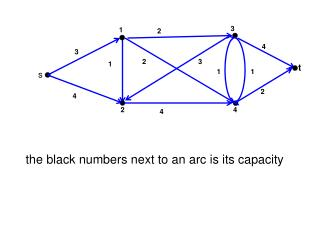DownloadDownload Presentation4

# 4

Télécharger la présentation## 4

- - - - - - - - - - - - - - - - - - - - - - - - - - - E N D - - - - - - - - - - - - - - - - - - - - - - - - - - -
##### Presentation Transcript

1. 3 1 2 4 3 2 3 1 t 1 1 s 2 4 2 4 4 the black numbers next to an arc is its capacity

2. 3 1 1 2 2 4 3 3 2 2 3 1 t 1 1 1 s 2 2 1 4 2 4 1 4 the black number next to an arc is its capacity the green number next to an arc is the flow on it extra 3 flow can be sent through (s,2) and then through (2,4); all arcs going out of 4 are saturated; but we can reroute 1 flow unit from (1,4) through (1,3) and then through (3,t), releasing 1 unit of flow through (4,t) which can then be used for extra flow on the (s,2)(2,4)(4,t)-path. To find such possibilities we do the bookkeeping in the so-called residual graph

3. 3 1 11 2 22 4 3 3 2 2 3 3 the residual graph 1 1 t 1 1 1 1 s 2 2 13 4 2 4 13 4 blue arcs (i,j) are forward arcs (fij<cij) green arcs (j,i) are backward arcs (fij>0) the blue number is the residual capacity of a blue arc the green number is the capacity of a green arc the black number is the original capacity of the arc

4. 3 1 11 22 3 2 3 the residual graph 1 t 1 1 s 2 13 2 4 13 red arcs form an augmenting path

5. 3 1 11 22 3 2 3 the residual graph 1 t 1 1 s 2 13 2 4 13 red arcs form an augmenting path Augment the flow by the minimum capacity of a red arc, i.e, 1

6. 3 1 2 3 1 3 3 the residual graph 1 t 11 1 1 s 2 22 2 4 22 red ars form an augmenting path Augment the flow by the minimum capacity of a red arc, i.e, 1 And construct the new residual graph

7. 3 1 2 4 2 2 3 1 t 1 1 s 2 4 2 4 4 An s-t cut is defined by a set S of the nodes with s in S and t not in S.

8. 3 1 2 4 2 2 3 1 t 1 1 s 2 4 2 4 4 S={s,1,2} An s-t cut is defined by a set S of the nodes with s in S and t not in S.

9. 3 1 2 4 2 2 3 1 t 1 1 s 2 4 2 4 4 S={s,1,2} An s-t cut is defined by a set S of the nodes with s in S and t not in S Size of cut S is the sum of the capacities on the arcs from S to N\S.

10. 3 1 2 4 2 2 3 1 t 1 1 s 2 4 2 4 4 C(S)=c13+c14+c24= 2+2+4=8 An s-t cut is defined by a set S of the nodes with s in S and t not in S Size of cut S is the sum of the capacities on the arcs from S to N\S.

11. S1 3 1 2 4 2 2 3 1 t 1 1 s 2 4 2 4 4 C(S1)=cs1+c24= 2+4=6

12. S2 S1 3 1 2 4 2 2 3 1 t 1 1 s 2 4 2 4 4 C(S1)=cs1+c24= 2+4=6 C(S2)=c13+c43+c4t= 2+1+2=5

13. S2 S1 3 1 2 4 2 2 3 1 t 1 1 s 2 4 2 4 4 C(S1)=cs1+c24= 2+4=6 C(S2)=c13+c43+c4t= 2+1+2=5 Max Flow ≤ Min Cut

14. S2 S1 3 1 2 4 2 2 3 1 t 1 1 s 2 4 2 4 4 C(S1)=cs1+c24= 2+4=6 C(S2)=c13+c43+c4t= 2+1+2=5 Max Flow ≤ Min Cut fs1+fs2 ≤ Min Cut ≤ 5

15. S2 1 2 3 1 3 3 the residual graph 1 t 11 1 1 s 2 22 2 4 22 = fs1+fs2=2+3=5 C(S2)=c13+c43+c4t= 2+1+2=5 S2={s,1,2,4} the set of nodes reachable from S Max Flow ≤ Min Cut

16. S2 1 2 3 1 3 3 the residual graph 1 t 11 1 1 s 2 22 2 4 22 = fs1+fs2=2+3=5 C(S2)=c13+c43+c4t= 2+1+2=5 S2={s,1,2,4} the set of nodes reachable from S Max Flow ≤ Min Cut Theorem: Max Flow = Min Cut